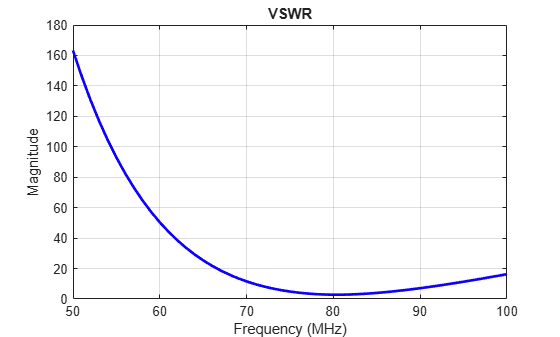# vswr

Voltage standing wave ratio (VSWR) of antenna or array element

## Syntax

``vswr(object,frequency,z0)``
``vswrant = vswr(object,frequency,z0)``

## Description

example

````vswr(object,frequency,z0)` calculates and plots the VSWR of an antenna or an array element, over specified frequency range, and a reference impedance of `z0` ohms.```

example

````vswrant = vswr(object,frequency,z0)` calculates and returns the VSWR of an antenna or array element, over specified frequency range, and a reference impedance of `z0` ohms.```

## Examples

collapse all

Plot vswr (voltage standing wave ratio) of a circular loop antenna.

```h = loopCircular; vswr(h,50e6:1e6:100e6,50)```Calculate vswr (voltage standing wave ratio) of a helix antenna.

```h = helix; hvswr = vswr(h,2e9:1e9:4e9,50)```
```hvswr = 1×3 3.6010 6.6936 3.3338 ```

## Input Arguments

collapse all

Antenna or array to calculate the VSWR, specified as an antenna or array from the catalog, custom antenna or array created using custom antenna or array objects in Custom 2-D and 3-D Antenna catalog, `pcbStack` antenna or array, or `installedAntenna`.

Frequency used to calculate VSWR, specified as a scalar in Hz or frequency range specified as vector in Hz.

Example: 75e6

Example: 50e6:1e6:100e6

Data Types: `double`

Reference impedance, specified as a scalar in ohms.

## Output Arguments

collapse all

VSWR, returned as a scalar in dB for a single analysis frequency and a vector in dB for a frequency range.

## Version History

Introduced in R2015a